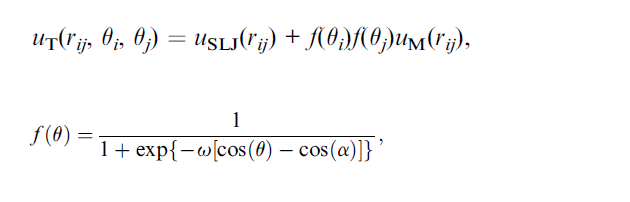# Anisotropic pairwise potential

Dear LAMMPS users,

I would like to ask if there is any possibility to employ this kind of potential that is enclosed to this message?

In the equation U_{SLJ} is shifted Lennard-Jones potential, U_M is the morse potential and f(\theta) are the orientation-dependent factors, and U_T is the total potential energy.
While the first two potentials are straightforward and easy to employ, however, is there any option to include these factors (2nd equation in the attached graphic) or it is necessary to

Sincerely,
Lukasz BaranDear LAMMPS users,

I would like to ask if there is any possibility to employ this kind of potential that is enclosed to this message?

In the equation U_{SLJ} is shifted Lennard-Jones potential, U_M is the morse potential and f(\theta) are the orientation-dependent factors, and U_T is the total potential energy.
While the first two potentials are straightforward and easy to employ, however, is there any option to include these factors (2nd equation in the attached graphic) or it is necessary to